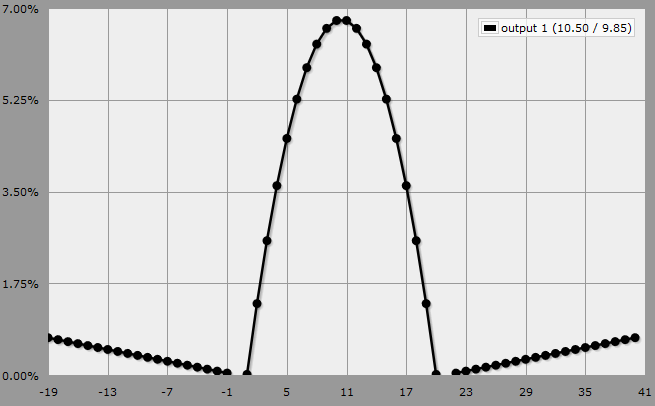# statistics – How to make Anydice see if two values are the same among 3 dice and keep them?Anydice is unfortunately a bit tricky in that it only allows us to return a single number from a function, so we have to use these workarounds if you want to get more information out of a single roll. But that’s entirely doable, in this case, since the introspection you want to do isn’t very complicated.

Here’s a program which implements your mechanic:

``````function: critical POOL:s {
if 1@POOL = 3@POOL { result: 2@POOL }
if 1@POOL = 2@POOL { result: 20 + 2@POOL }
if 2@POOL = 3@POOL { result: 2@POOL - 20 }
result: 2@POOL
}

output [critical 3d20]
``````

We invoke the function `critical` with a 3d20 roll, which is cast to a sequence in descending order. Stepping through the function:

1. If the highest die and the lowest die are equal, we must have a triple, which I assume cancels out and we just take the value of the middle die.
2. If the highest die equals the middle die, we have a positive critical and we add 20 to the result to indicate that.
3. If the middle die equals the lowest die, we have a negative critical and we subtract 20 from the result to indicate that.
4. Otherwise, we just take the value of the middle die.

The resulting output shows the probability curve you’d expect for taking the middle die of a 3d20 roll, but with a couple of wings either side that represent the critical rolls. Note that there is zero probability of getting a result of 0 or 21, because it’s impossible to get a negative crit if the middle die is a 20 and impossible to get a positive crit if the middle die was a 1.